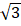# At a given instant, say t = 0, two radioactive substance A and B have equal activities.  The ratioof their activities after time t itself decays with time t as e−34 .  If the half-life of A is m2 , the half-life of B is a.b. 2ln2 c.d. 4ln2

## Question ID - 50110 :- At a given instant, say t = 0, two radioactive substance A and B have equal activities.  The ratioof their activities after time t itself decays with time t as e−34 .  If the half-life of A is m2 , the half-life of B is a.b. 2ln2 c.d. 4ln2

3537

Half life of A = ln2

t1/2 =λA = 1

at  t = 0 RA = RB

Ne−λAT = NB e−λBT

N= NB     at  t = 0

at t = t== e−t

λB−λA = 3

λB= 3 + λA = 4

t1/2 ==Next Question :
 From a point within an equilateral triangles perpendiculars drawn to the three sides are 6 cm, 7 cm and 8 cm. the length of the side of the triangle is (a) 7 cm (b) 10.5 cm (c) 14cm (d)cm